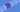# ES6: The Let Keyword

### 2019-07-13

In ES6, the `let` keyword was introduced to address some of the issues with the `var` keyword. Let's take a look at the differences.

First of all, note that the `let` keyword is considered to be scoped to the block. The examples illustrate this.

## Global

When using `let` in the global scope (in a file outside of functions), it works similar to the `var` keyworkd.

``````var count = 0;
let number = 1;``````

Yet, when using in a browser, the `var` will attach to the window, but not the `let`.

``````window.count // equals 0
window.number // undefined``````

## Function

`let` and `var` work the same inside a function.

``````function myCoolFunction() {
let myLetVariable = 'woot';
var myLetVariable = 'woot, woot';
}``````

## Block or For Loops

The big difference is when using `var` and `let` insidee of a For Loop.

``````functon forLoopWithLet() {
// can't access count

for (let count = 0; count < 10; count += 1) {
// can access count and each count is a new variable
}

// can't access count
}

functon forLoopWithVar() {
// you can access count

for (var count = 0; count < 10; count += 1) {
// can access count and each count is the same variable
}

// you can access count
}``````

## Declaring

The final difference is that `var` variablees can be redeclared, but `let` can not.

``````var one = 'one';
var one = 'two'; // Works``````
``````let one = 'one';
let one = 'two'; // Syntax Error``````